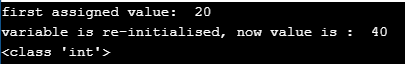# Variables in Python

## Variables in Python with Examples

In this article, I am going to discuss Variables in Python with Examples. Please read our previous article where we discussed Naming Conventions in Python. At the end of this article, you will understand the following pointers in detail.

1. What is a variable in python?
2. Properties of variable
3. Creating variable
4. Invalid cases for variables
5. Multiple variables in n single line
6. Single value for multiple
7. Variable re-initialization
8. Multiple Programs to understand the above concepts
##### What is a variable in Python?

All the data which we create in the program will be saved in some memory location on the system. The data can be anything, an integer, a complex number, a set of mixed values, etc.

If we want to use the data, which is saved in the memory location, we need to call the reference of that location, which we don’t know. What if we give some name of our choice to that memory location? This will help us in using that data by referring to the name which we have given to that memory location. That name is called variable.

Variable is the name that we give to the memory location which holds some data. With a variable, we can store the data, access the data, and also manipulate the data. We can also refer to variables as containers which store some information/data.

###### To summarize, a variable is a
1. Name
2. Refers to a value
3. Hold some data
4. Name of a memory location

Example 1: (printing variable)
Code:
emp_id = 11
print(emp_id)
Output: 11

##### Properties of a variable in Python:

Every variable has some properties which each programmer should have some basic idea of.

Type: Each and every programming language has data types and each variable should belong to one of the data types. This is called the type of variable. In Python language, the data types available are lists, tuples, dictionaries, strings, sets, etc which will be explained in the later chapters in detail.

Scope: In any programming language, scope refers to the place or limit or boundaries or the part of the program within which the variable is accessible. Generally, the two types of scopes defined are the global scope and local scope which will be discussed in later chapters.

Value: Each and every variable in a program holds the value of some type that can be accessed or modified during the due flow of the program.

Location: As already stated, the data is stored in the memory location. The variable is the name that we give to that location. Each memory location has some address associated with it.

Lifetime: The lifetime of a variable refers to the time period for which the memory location associated with the variable stores the data.

##### Creating a variable in Python

Generally, in many programming languages, while defining or creating a variable, we need the data type and variable name and value. For example, in C language variable creation

int i = 10;

Python is a dynamically typed programming language. There is no need of specifying the data type for a variable. The program automatically takes the data type dynamically during the creation. In python, to create a variable we just need to specify

1. Name of the variable
2. Assign a value to the variable

Syntax to create variable in python: name_of_the_variable = value

Code:
age = 110
print(age)
Output: 110

###### Example 3: (creating a variable and printing with a beautiful message)

Code:
age = 16
print(“My age is sweet”, age)
Output: My age is sweet 16

Note: We can print meaningful text messages along with variables for better understanding.

1. Text message we should keep in within double-quotes.
2. Text message and variable name should be separated by comma symbol.
##### Invalid cases for variables:

While defining variables in python,

1. Variable names should be on the left side.
2. The value should be on the right side.
3. Violating this will result in a syntax error

Example 4: (creating variable in wrong direction)
Code:
10 = emp_id
print(emp_id)
Output: SyntaxError: can’t assign to literal

Variable names should not be keywords, else will result in an error.

Example 5: (creating a variable with keyword name)
Code:
if = 10
print(if)
Output: SyntaxError: invalid syntax

##### Multiple variables in a single line in Python:

We can assign multiple variables to multiple values in a single line. During the assignment, the variable name on the left-hand side should be equal to the values on the right-hand side. If not it results in an error.

Example 6: (assigning multiple variables in single line)
Code:
a,b,c = 10,20,30
print(a,b,c)
Output: 10 20 30

Example 7: (unequal items on either side)
Code:
a,b,c = 10,20
print(a,b,c)
Output: ValueError: not enough values to unpack (expected 3, got 2)

Example 8: (unequal items on either side)
Code:
a,b = 10,20,30
print(a,b,c)
Output: ValueError: too many values to unpack (expected 2)

##### Single value for multiple variables in Python

We can assign a single value to multiple variables simultaneously.

Example 9: (assign value to multiple variables)
Code:
a = b = c = 10
print(a,b,c)
Output: 10 10 10

##### Re-initialize the variables in Python

We can reinitialize the variable values. The old values will be overridden or replaced with the new values.

Example 10: (re-initializing the variables)
Code:
a = 20
print(“first assigned value: “, a)
a = 40
print(“variable is re-initialised, now value is : “, a)
print(type(a))
Output:Note: Python has an in-built function called type() which gives the datatype of the variable which is defined in the program. In the above example, since the variable ‘a’ is of ‘int’ data type so type(a) gives the output as ‘int’.